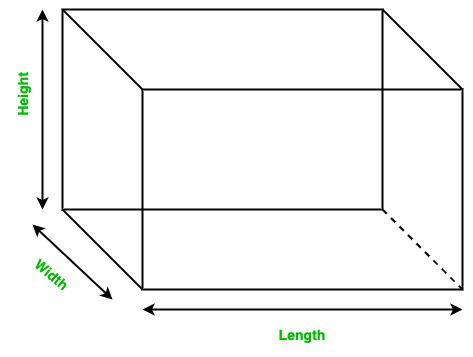Open in App
Not now

# What happens to the surface area of cuboid, if its length, breadth and height is doubled?

• Last Updated : 28 Nov, 2021

Mensuration is a branch of mathematics that deals with the study of geometric figures and parameters such as volume, area, surface area, etc. It can ideal with both 2-D and 3-D figures. Or we can say that, when we are working with the area, the volume of specific shapes, or different parameters of geometric figures then it is called Mensuration in Mathematics.

Cuboid

A cuboid is a three-dimensional geometrical figure, enclosed within 6 faces. It is recognized using three dimensions, namely, length, breadth, and height. It can be visualized as a stack of rectangular sheets joined together. All the vertices of a cuboid are 90°.Surface Area of Cuboid

The surface area of the cuboid, denoted by S.A is equivalent to the summation of the area of all the six faces of the cuboid. Since the product of any rectangular face is equal to the product of any two dimensions, therefore, S.A is given by,

Surface Area = 2lw + 2lh + 2hw

S.A = 2 (lw + lh + hw)

Here, S.A is the surface area of the cuboid, l be the length, h be the height, and w be the width of the cuboid. Thus, the surface area is equivalent to the product of lengths, taken two at a time. The surface area of the cuboid is, therefore, measured in terms of square units.

### What happens to the surface area of cuboid, if its length, breadth and height is doubled?

Solution:

As we know that

S.A = 2 (lw + lh + hw)

Let us assume l’ , w’ and h’ to be the new length, width and height respectively. Also, the new surface area to be denoted by S.A’.

According to the problem statement,

SA’ =  2 (l’w’ + l’h’ + h’w’)

Now, each of the length, width and height  are doubled, thereby,

l’ = 2l

h’ = 2h

w’ = 2w

S.A’ = 2 (2l x 2w + 2l x 2h + 2h x 2w)

SA’ = 2 x 4 (lw + lh + hw)

On solving,

S.A’ = 4 x 2 (lw + lh + hw)

Since,

S.A = 2 (lw + lh + hw)

So,

S.A’ = 4 x S.A

The surface area of cuboid therefore becomes four times if we double all the dimensions of the cuboid.

### Similar Questions

Question 1: Can you derive the surface area of the cube using the surface area of the cuboid?

Solution:

Since, we know,

Surface Area of Cuboid = 2 (lw + lh + hw), where let us assume the length to be denoted by l, height to be denoted by h and width to be denoted by w respectively.

In case of a cube, length, width and height of a cube are equivalent. Let us assume ‘a’ to be the length, breadth and height of the cube.

On substituting the values, we obtain,

Surface Area of cube = 2 (a x a + a x a + a x a)

= 2 (3 a2)

= 6 a2

Question 2: Using the above formula, find the surface area of cuboid, with length, breadth and height each equal to 2m.

Solution:

Surface Area of cube = 6 a2

We have, a = 2m

Therefore,

Surface Area = 6 x 22

= 6 x 4 m2

= 24 m2

Question 3: What happens to the surface area of cuboid if the length is made half of the original?

Solution:

As we know that,

S.A = 2 (lw + lh + hw)

Now,

New surface area, SA’ =  2 (l’w’ + l’h’ + h’w’)

l’ = 1/2l

w’ = w

h’ = h

SA’ = 2(1/2l x w + 1/2 l x h + h w)

SA’ = 2(1/2 (lw + lh) + hw)

SA’ = lw + lh + 2hw

Question 4: Derive the formula if length, breadth, and height of cuboid of are made n times each.

Solution:

As we know that,

S.A = 2 (lw + lh + hw)

Now,

New surface area, SA’ =  2 (l’w’ + l’h’ + h’w’)

l’ = nl

h’ = nh

w’ = nw

SA’ = 2 (nl x nw + nl x nh + nh x nw)

SA’ = 2n2 (lw + lh + hw)

SA’ = n2 x SA

Therefore, the surface area becomes n2 times.

Question 5: Using the above formula, indicate how the surface area changes in case each dimension is reduced to 1/3 times.

Solution:

The surface area becomes (1/3)2 times.

Therefore,

The new surface area = 1/9 times the original surface area.

My Personal Notes arrow_drop_up